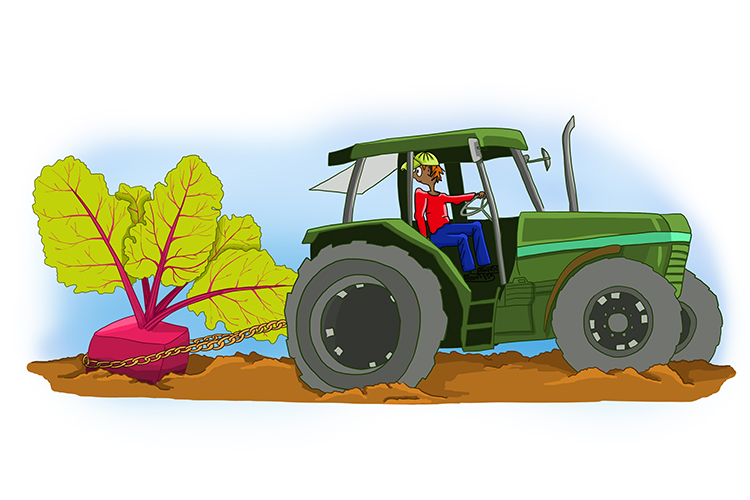# Surd

## Can't remove the square root (unsolvable square root)

A surd is a number that can’t be simplified to remove a square root.“That’s absurd! (surd) Surely you can remove a square beetroot.” (Square root). But they couldn’t remove it (can’t remove).

Examples

1.   sqrt2 can’t be removed, therefore sqrt2 is a surd.

2.   sqrt4 is equal to 2, so it can be removed, therefore sqrt4 is not a surd.

## Further surds – Always think sqrt9

If you see the term surds in an exam you must write out the following.

Surds - Always think sqrt9

Try something you know.

As       sqrt9+sqrt9=3+3=6

See if  sqrt9+sqrt9=2sqrt9 also equals 6

=2times3 does equal 6

Therefore sqrt9+sqrt9=2sqrt9

## Subtraction

sqrt9-sqrt9=3-3=0

## Multiplication

sqrt9timessqrt9=3times3=9=sqrt81=sqrt(9times9)

sqrt9timessqrt9=sqrt(9times9)

## Divide

sqrt9/sqrt9=3/3=1=sqrt(9/9)

sqrt9/sqrt9=sqrt(9/9)

Now you can work out any surd question.